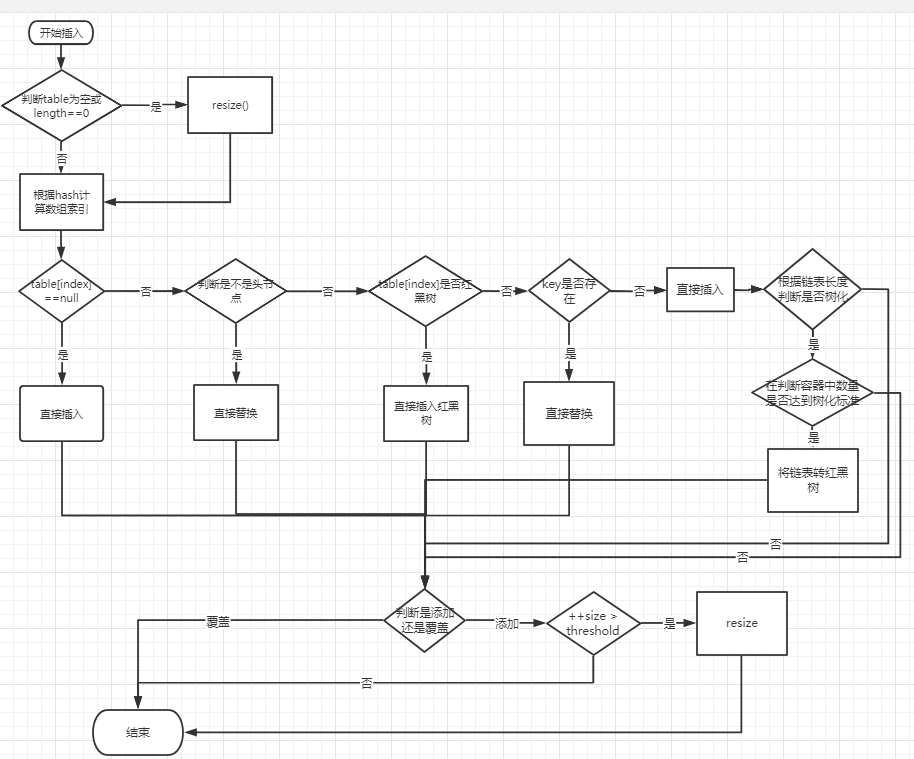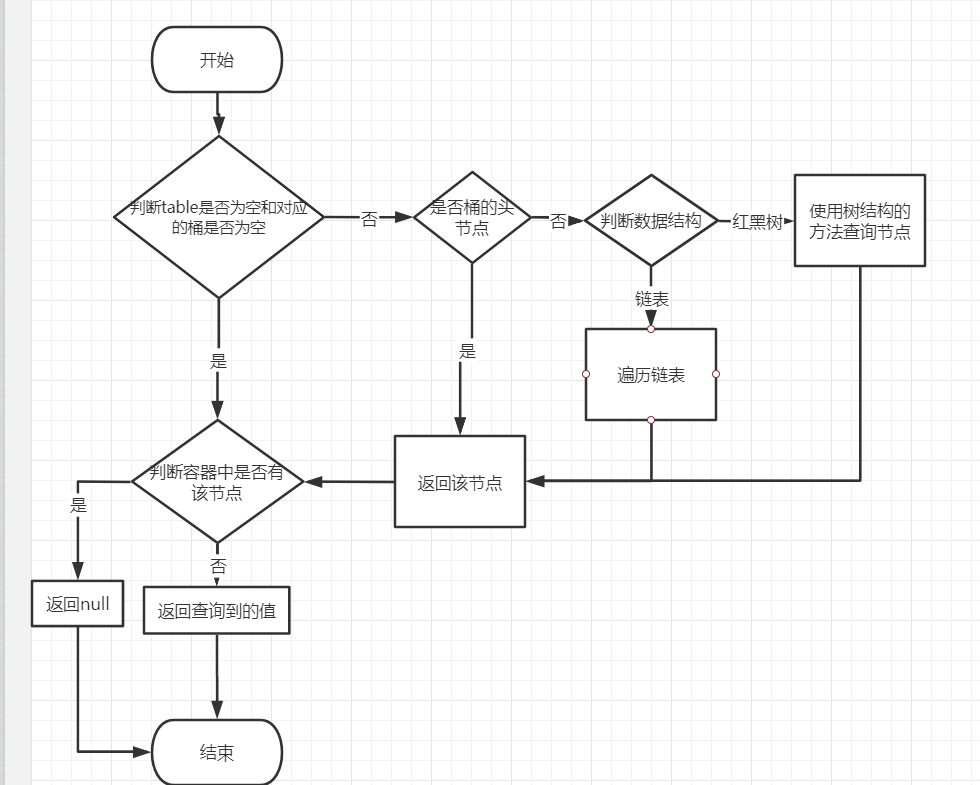# 正文

## 1.8版本

### 构造方法

 `1234567891011121314151617181920212223` `````` // 无参构造方法 public HashMap() { this.loadFactor = DEFAULT_LOAD_FACTOR; // all other fields defaulted } // 指定容器初始化数组容量 public HashMap(int initialCapacity) { this(initialCapacity, DEFAULT_LOAD_FACTOR); } // 指定容器的初始化数组的容量 public HashMap(int initialCapacity, float loadFactor) { if (initialCapacity < 0) throw new IllegalArgumentException("Illegal initial capacity: " + initialCapacity); if (initialCapacity > MAXIMUM_CAPACITY) initialCapacity = MAXIMUM_CAPACITY; if (loadFactor <= 0 || Float.isNaN(loadFactor)) throw new IllegalArgumentException("Illegal load factor: " + loadFactor); this.loadFactor = loadFactor; // 将传入的值赋予经过tableSizeFor后赋值给threshold； this.threshold = tableSizeFor(initialCapacity); } ``````

1. 这里并没有一开始就初始化容器中的数组，只是将传入的数量赋值给threshold；
2. 还需要注意的是tableSizeFor这个方法，这个方法会自动将传入的值改变为一个为2整数次幂的一个整数；

### 添加方法

 `12345` `````` public V put(K key, V value) { return putVal(hash(key), key, value, false, true); } ``````

1. 注意这里的hash方法，key为null会返回0，HashMap可以存null 的key就是在这里体现的；

 `123456789101112131415161718192021222324252627282930313233343536373839404142434445464748495051525354555657` `````` final V putVal(int hash, K key, V value, boolean onlyIfAbsent,boolean evict) { Node[] tab; Node p; int n, i; // 判断当前容器数组是否已经初始化 if ((tab = table) == null || (n = tab.length) == 0) // 这里是初始化容器数组 n = (tab = resize()).length; // 判断数组对应索引位置是否有数据，无则直接将存入的数作为链头放入数组 if ((p = tab[i = (n - 1) & hash]) == null) tab[i] = newNode(hash, key, value, null); else { Node e; K k; // 判断存入对象是否与该位置链表的头节点一致 if (p.hash == hash &&((k = p.key) == key || (key != null && key.equals(k)))) e = p; // 判断对象存入对应位置的数据结构是否为红黑树 else if (p instanceof TreeNode) // 存入红黑树 e = ((TreeNode)p).putTreeVal(this, tab, hash, key, value); else { // 循环对应位子的链表 for (int binCount = 0; ; ++binCount) { if ((e = p.next) == null) { // 链表末尾添加该节点 p.next = newNode(hash, key, value, null); // 判断是否要将链表转红黑树 if (binCount >= TREEIFY_THRESHOLD - 1) // -1 for 1st // 将链表转红黑树 treeifyBin(tab, hash); break; } // 判断链表中是否存在该对象，存在则替换 if (e.hash == hash && ((k = e.key) == key || (key != null && key.equals(k)))) break; p = e; } } // 判断链表中是否存在存入的对象 if (e != null) { // existing mapping for key V oldValue = e.value; if (!onlyIfAbsent || oldValue == null) e.value = value; afterNodeAccess(e); // 返回原来的值 return oldValue; } } ++modCount; // 判断存入对象后是否达到扩容标准 if (++size > threshold) // 这里是扩容 resize(); afterNodeInsertion(evict); return null; } ``````

1. 注意resize方法，该方法实现将容器真正的初始化和扩容
2. 容器中的红黑树是用TreeNode实现的
3. 这里的链表插入是插入末尾
4. 判断是否将链表转为红黑树是根据TREEIFY_THRESHOLD这个属性来判断的，转换调用的方法是treeifyBin

### resize方法

 `123456789101112131415161718192021222324252627282930313233343536373839404142434445464748495051525354555657585960616263646566676869707172737475767778798081828384858687888990` `````` final Node[] resize() { Node[] oldTab = table; int oldCap = (oldTab == null) ? 0 : oldTab.length; int oldThr = threshold; int newCap, newThr = 0; // 判断是否扩容 if (oldCap > 0) { // 判断数组是否达到最大值 if (oldCap >= MAXIMUM_CAPACITY) { threshold = Integer.MAX_VALUE; return oldTab; } // 扩容一倍，变为原来两倍 else if ((newCap = oldCap << 1) < MAXIMUM_CAPACITY && oldCap >= DEFAULT_INITIAL_CAPACITY) newThr = oldThr << 1; // double threshold } // 判断是否在构造时是否指定了容量 else if (oldThr > 0) // initial capacity was placed in threshold newCap = oldThr; else { // zero initial threshold signifies using defaults // 没指定就使用默认值，数组初始化长度为DEFAULT_INITIAL_CAPACITY16 newCap = DEFAULT_INITIAL_CAPACITY; // 将容器扩容标准设置为默认值（初始化长度*加载因子；就是数组长度的3/4） newThr = (int)(DEFAULT_LOAD_FACTOR * DEFAULT_INITIAL_CAPACITY); } // 如果构造容器时指定了容量，则会将扩容标准设置为指定值的3/4 if (newThr == 0) { float ft = (float)newCap * loadFactor; newThr = (newCap < MAXIMUM_CAPACITY && ft < (float)MAXIMUM_CAPACITY ? (int)ft : Integer.MAX_VALUE); } threshold = newThr; @SuppressWarnings({"rawtypes","unchecked"}) // 构造扩容后的新数组 Node[] newTab = (Node[])new Node[newCap]; table = newTab; // 判断原来的数组中是否有数据 if (oldTab != null) { // 循环原来数组中的每个节点 for (int j = 0; j < oldCap; ++j) { Node e; // 判断空 if ((e = oldTab[j]) != null) { oldTab[j] = null; // 如果该节点只有一个对象，则直接放入新的数组中对应的位置 if (e.next == null) newTab[e.hash & (newCap - 1)] = e; // 判断该节点是否为红黑树 else if (e instanceof TreeNode) // 将红黑树拆分，并放入新的数组中 ((TreeNode)e).split(this, newTab, j, oldCap); else { // preserve order Node loHead = null, loTail = null; Node hiHead = null, hiTail = null; Node next; // 将该节点的链表拆分，拆分后只会放入新的数组中的两个节点中 do { next = e.next; if ((e.hash & oldCap) == 0) { if (loTail == null) loHead = e; else loTail.next = e; loTail = e; } else { if (hiTail == null) hiHead = e; else hiTail.next = e; hiTail = e; } } while ((e = next) != null); if (loTail != null) { loTail.next = null; newTab[j] = loHead; } if (hiTail != null) { hiTail.next = null; newTab[j + oldCap] = hiHead; } } } } } return newTab; } ``````

1. 原来数组中的数据放到新数组中，只会存在两个节点中；
2. 扩容是变为原来的两倍，是数组长度扩容

### treeifyBin 方法

 `12345678910111213141516171819202122232425` `````` final void treeifyBin(Node[] tab, int hash) { int n, index; Node e; // 判断数组长度是否达到树化的标准MIN_TREEIFY_CAPACITY=64 if (tab == null || (n = tab.length) < MIN_TREEIFY_CAPACITY) resize(); // 遍历链表，将其转换为树，注意并不是红黑树 else if ((e = tab[index = (n - 1) & hash]) != null) { TreeNode hd = null, tl = null; do { TreeNode p = replacementTreeNode(e, null); if (tl == null) hd = p; else { p.prev = tl; tl.next = p; } tl = p; } while ((e = e.next) != null); if ((tab[index] = hd) != null) // 这里转换红黑树 hd.treeify(tab); } } ``````

1. 链表转红黑树并不是链表长度达到8之后就可以，而是还需要数组长度也达到64以上

### get方法

 `123456` `````` public V get(Object key) { Node e; // 判断容器中是否有该节点，有返回value，无则返回null return (e = getNode(hash(key), key)) == null ? null : e.value; } ``````

### getNode方法

 `1234567891011121314151617181920212223242526` `````` final Node getNode(int hash, Object key) { Node[] tab; Node first, e; int n; K k; // 判断数组长度以及对应索引的桶是否也为空 if ((tab = table) != null && (n = tab.length) > 0 && (first = tab[(n - 1) & hash]) != null) { // 判断是否桶中第一个节点 if (first.hash == hash && // always check first node ((k = first.key) == key || (key != null && key.equals(k)))) return first; // 判断是否还有下一个节点 if ((e = first.next) != null) { // 判断是否为树 if (first instanceof TreeNode) // 按树的结构来查找 return ((TreeNode)first).getTreeNode(hash, key); do {// 遍历链表查找 if (e.hash == hash && ((k = e.key) == key || (key != null && key.equals(k)))) return e; } while ((e = e.next) != null); } } return null; } ``````

### put流程图### get流程图## 1.7与 1.8比较

1. 数据结构
1.7使用数组加链表的数据结构，1.8使用数组，链表加红黑树的数据结构

2. 初始化以及扩容
1.7使用

3. 数据插入方式
1.7使用头插法，将数据插入头节点；1.8使用尾插法，将数据插入链表末尾，即便是扩容也是尾插法

4. hash计算方式
1.8使用了一次位运算加一次异或运算；1.7进行了四次位运算加五次异或运算；